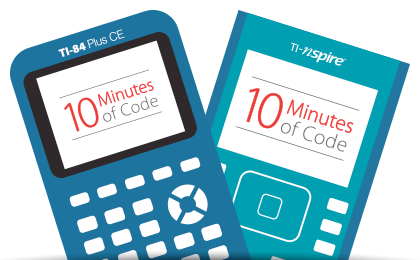# Code by Numbers - A STEM module based on number

Coding, pseudo-coding, problem solving, investigations and a wealth of mathematical discovery and understanding - Code by Numbers. This module consists of a sequence of four activities, ideally suited for a middle school mathematics elective. Download the complete series by selecting the appropriate link below, or check out each individual activity.

## Counting Factors

Students develop a rule for the quantity of factors for any number based on its prime factorisation.

## Euclid's Algorithm

Turn one of the oldest mathematical algorithms into a functioning program and then use it to efficiently explore a list of numbers and more.

## Euler Totient Function

It sounds complicated, it's not. The Euler Totient function counts co-prime numbers to the selected number. So many patterns emerge as students determine the ETF for a set of numbers.

## Highly Composite

Studied by the famous mathematician: Ramanujan, Highly Composite Numbers are still be explored today.

## Lessons

### Develop skills for 21st-century success with free project-based learning activities that help students explore, understand and connect the principles of science, math, coding, engineering design and electronics.## 10 Minutes of Code

Introduce students to the basics of coding and build their understanding of math with simple, 10-minute lessons for their TI-84 Plus CE or TI-Nspire™ CX graphing technology.